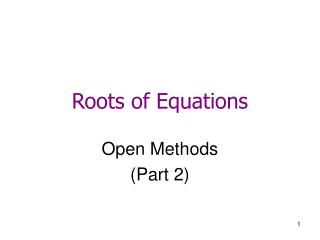# Roots of Equations - PowerPoint PPT PresentationDownload PresentationRoots of Equations

Download Presentation## Roots of Equations

- - - - - - - - - - - - - - - - - - - - - - - - - - - E N D - - - - - - - - - - - - - - - - - - - - - - - - - - -
##### Presentation Transcript

1. Roots of Equations Open Methods (Part 2)

2. The following root finding methods will be introduced: A. Bracketing Methods A.1. Bisection Method A.2. Regula Falsi B. Open Methods B.1. Fixed Point Iteration B.2. Newton Raphson's Method B.3. Secant Method

3. B.2. Secant Method Newton-Raphson method needs to compute the derivatives. The secant method approximate the derivatives by finite divided difference. From Newton-Raphson method

4. Secant Method

5. Secant Method – Example Find root of f(x) = e-x - x = 0 with initial estimate of x-1 = 0 and x0 = 1.0. (Answer: α= 0.56714329) Again, compare this results obtained by the Newton-Raphson method and simple fixed point iteration method.

6. Comparison of the Secant and False-position method • Both methods use the same expression to compute xr. • They have different methods for the replacement of the initial values by the new estimate. (see next page)

7. Comparison of the Secant and False-position method

8. Comparison of the Secant and False-position method

9. Modified Secant Method • Needs only one instead of two initial guess points • Replace xi-1 - xi by δxi and approximate f'(x) as • From Newton-Raphson method,

10. Modified Secant Method Find root of f(x) = e-x - x = 0 with initial estimate of x0 = 1.0 andδ=0.01. (Answer: α= 0.56714329) Compared with the Secant method

11. Modified Secant Method – About δ If δ is too small, the method can be swamped by round-off error caused by subtractive cancellation in the denominator of If δ is too big, this technique can become inefficient and even divergent. If δ is selected properly, this method provides a good alternative for cases when developing two initial guess is inconvenient.

12. The following root finding methods will be introduced: A. Bracketing Methods A.1. Bisection Method A.2. Regula Falsi B. Open Methods B.1. Fixed Point Iteration B.2. Newton Raphson's Method B.3. Secant Method Can they handle multiple roots?

13. Multiple Roots • A multiple root corresponds to a point where a function is tangent to the x axis. • For example, this function has a double root. f(x) = (x – 3)(x – 1)(x – 1) = x3 – 5x2 + 7x - 3 • For example, this function has a triple root. f(x) = (x – 3)(x – 1)(x – 1) (x – 1) = x4 – 6x3 +12x2 - 10x + 3

14. Multiple Roots • Odd multiple roots cross the axis. (Figure (b)) • Even multiple roots do not cross the axis. (Figure (a) and (c))

15. Difficulties when we have multiple roots • Bracketing methods do not work for even multiple roots. • f(α) = f'(α) = 0, so both f(xi) and f'(xi) approach zero near the root. This could result in division by zero. A zero check for f(x) should be incorporated so that the computation stops before f'(x) reaches zero. • For multiple roots, Newton-Raphson and Secant methods converge linearly, rather than quadratic convergence.

16. Modified Newton-Raphson Methods for Multiple Roots • Suggested Solution 1: Disadvantage: work only when m is known.

17. Modified Newton-Raphson Methods for Multiple Roots • Suggested Solution 2:

18. Example of the Modified Newton-Raphson Method for Multiple Roots • Original Newton Raphson method The method is linearly convergent toward the true value of 1.0.

19. Example of the Modified Newton-Raphson Method for Multiple Roots • For the modified algorithm

20. Example of the Modified Newton-Raphson Method for Multiple Roots • How about their performance on finding the single root?

21. Modified Newton-Raphson Methods for Multiple Roots • What's the disadvantage of the modified Newton-Raphson Methods for multiple roots over the original Newton-Raphson method? • Note that the Secant method can also be modified in a similar fashion for multiple roots.

22. Summary of Open Methods • Unlike bracketing methods, open methods do not always converge. • Open methods, if converge, usually converge more quickly than bracketing methods. • Open methods can locate even multiple roots whereas bracketing methods cannot. (why?)

23. Study Objectives • Understand the graphical interpretation of a root • Understand the differences between bracketing methods and open methods for root location • Understand the concept of convergence and divergence • Know why bracketing methods always converge, whereas open methods may sometimes diverge • Realize that convergence of open methods is more likely if the initial guess is close to the true root.

24. Study Objectives • Understand what conditions make a method converges quickly or diverges • Understand the concepts of linear and quadratic convergence and their implications for the efficiencies of the fixed-point-iteration and Newton-Raphson methods • Know the fundamental difference between the false-position and secant methods and how it relates to convergence • Understand the problems posed by multiple roots and the modifications available to mitigate them